Numerical Computation of Net Radiative Heat Transfer within a Non Absorbing Furnace Enclosure

Shuaibu Ndache MOHAMMED

Department of Mechanical Engineering, Federal University of Technology, Minna, Nigeria

Abstract

The numerical evaluation of the net radiative heat transfer rate in a single zone, non absorbing furnace enclosure is reported. In this analysis, simplified mathematical furnace model namely, the long furnace model is used to determine furnace performance. The formulation assumes some known temperature values. Thus, heat transfer equations were set up and solved numerically. A FORTRAN computer program was developed and debugged. Results obtained from this study compare favourably well with the results from the traditional graphical method. Also, the computer program developed can handle variations in furnace operating conditions, temperatures, thermal properties and dimensions.

Keywords

Numerical, Radiative, Furnace, Zone, View factor, Heat transfer, Temperature

Introduction

Furnaces have wide applications in engineering, with the main function of heating materials, often known as stock or charge, to a predetermined temperature and enthalpy.

Furnaces are used extensively especially in the steel making industry. The heat required for the process may be produced either by the combustion of a fuel or electrically by resistive, inductive, capacitative or arc heating (Gray and Muller, 1974).

Furnaces are commonly classified into batch and continuous. In the batch furnace, the stock placed in the enclosure, heated to the required temperature and the removed. While, in the continuous furnace, the stock is moved progressively through the enclosure as it is heated (Kreith, 1976). Figure 1 shows a typical furnace geometry used for the analysis.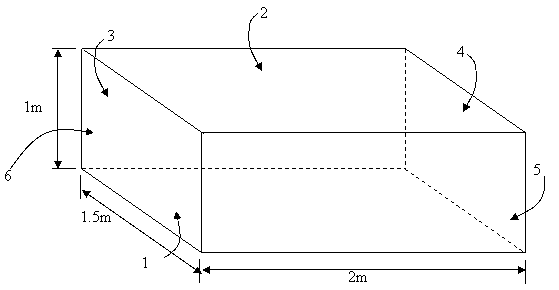Figure 1 Rectangular Furnace Geometry

Bevans and Dunkle (1960) carried out extensive work on the evaluation of radiant interchange within an enclosure. They setup all the radiant heat transfer equations and solved them by simple numerical technique.

Kreith in 1962 presented data on view factors for some geometry in chart form. Sparrow (1973) presented more data on view factors based on the analytical intergration of the view factor integral equation for a large number of geometrical configurations. More information on the evaluation of view factors between parallel plates and touching rectangular plates were presented by Hottel and Sarofin in 1967.

Theoretical background

The assumption that energy exchange by radiation is the predominant heat-flow mechanism at high temperatures because the rate of heat flow depends on the fourth power of the absolute temperature is employed (Kreith, 1976). The equations of the radiative heat transfer rates that are used in the analysis follows:

The process by which heat is transferred from a body by virtue of its temperature, without the aid of any intervening medium is the thermal radiation, which travels at the speed of light (3 x 108 m/s) in a vacuum. A heated body loses energy continuously by radiation, at a rate dependent on the shape, size, and particularly, the temperature of the body (Kreith, 1976).

The direct exchange of radiation between two surfaces depends on two factors:

·      The view which the surfaces have of each other;

·      The emitting and absorbing characteristics of the surfaces.

The view factor from one surface to another is defined as the fraction of the total radiation emitted by the one surface which is directly incident on the other. Thus, from Figure 2, the radiation leaving surface 1 and directly incident upon surface 2 is:

Q = ε1A1Eb1F12                                                                                                (1)

where F12 is the view factor from surface 1 to 2.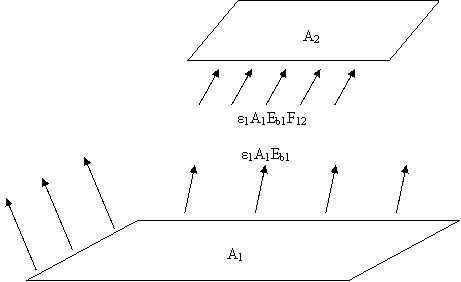Figure 2. Direct transmission of radiation from one surface to another

The summation of all view factors from a surface to all other surfaces receiving radiation from it is 1. Thus, for an enclosure comprising n surfaces:

F11 + F12 + F13 + … + F1n = 1                                                                          (2) ∑F1j = 1                                                                                                     (3)

The key problem in calculating radiation heat transfer between surfaces is to determine the view factor Fij. In general, however, the determination of view factors for surfaces is usually difficult especially for complex configurations. View factors are evaluated from the following expression

Fij = (1/Ai)∫Ai∫Aj (cosΦicosΦjdAidAj)/(πr2)                                                                   (4)

Algebraic expressions from which the view factors can be computed for the surface configurations shown schematically in Figures 3(a) and (b) are as follows:

Configuration (a): Identical, parallel rectangular plates (Figure 3a)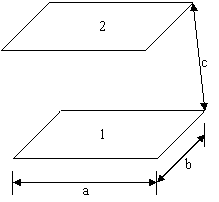Figure 3a. Identical, Parallel Rectangular Plates

X = a / c                                                                                                           (5)

Y = b / c                                                                                                          (6)

FA1-A2(πXY/2) = ℓn[(1 + X2)(1 + Y2)/(1 + X2 + Y2)]1/2

+Y√(1 + X2)tan-1[Y/√(1 + X2)]

+ X√(1 + Y2)tan-1[X/√(1 + Y2)]

- Y tan-1Y - X tan-1X                                                                (7)

Configuration (b): Touching inclined plates (Figure 3b)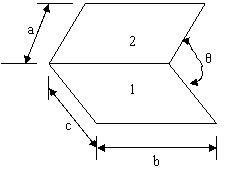Figure 3b. Touching Inclined Plates

X = a / b                                                                                                          (8)

Y = c / b                                                                                                          (9)

Z = X2 + Y2 - 2XYCosθ                                                                                  (10)

FA1-A2(πY) = -Sin2θ[XYSinθ +(π/2 - θ)(X2 +Y2) +Y2 tan-1{(X-YCosθ)

/(YSinθ)}+ X2 tan-1{(Y-XCosθ)/(XSinθ)}]/4

+ Sin2θ [(2/Sin2θ - 1) x ℓn{(1 + X2)(1 + Y2)/(1 + Z)}

+ Y2ℓn{Y2 (1+Z)/((1 + Y2)Z)} + X2ℓn{(X2(1+X2)Cos2θ)

/((1 + Z)ZCos2θ)}+ Y tan-1(1/Y) + Xtan-1(1/X) - √Z tan-1(1/√Z)

+ Sinθ Sin2θ/2x√(1 + X2 Sin2θ)[{tan-1(XCosθ/√(1+X2 Sin2θ)}

+tan-1{(Y-XCosθ)/√(1+X2Sin2θ)}]+Cosθ∫Y0√(1+ξ2Sin2θ)

[tan-1{(X-ξCosθ)/√(1+ξ2Sin2θ)}+tan-1{ξCosθ/√(1+ξ2Sin2θ)]dξ            (11)

Radiative Heat Transfer in an Empty Enclosure

Consider an enclosure comprising n surfaces at temperatures T1, T2, T3, …, Tn (see Figure 4). The total radiant heat transfer from a surface i is given by:

Wi = εiEbi + ρiHi                                                                                              (12)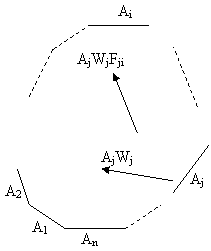Figure 4. Enclosure Comprising of n Surfaces

An amount of radiation (AjWj) leaves every surface of area Aj and a fraction of this Fji is directly incident upon surface i. Thus, the total energy incident upon surface i is the sum of terms like AjWjFji (see equation 13 that follows):

AiHi = W1A1F1i +W2A2F2i + … + WnAnFni                                                     (13)

Since AiFij = AjFji, we find

AiHi = W1AiFi1 + W2AiFi2 + … + WnAiFin                                                      (14)

or

Hi = ∑WjFij                                                                                                     (15)

Substituting equation (15) into (12) leads to

Wi = = εiEbi + ρi∑WjFij                                                                                   (16)

or

Ai ∑WjFij -  AiWii = -Ai εi Ebii                                                                   (17)

where i = 1, 2, …, n.

An equation of this kind produced for each surface and provides n linear simultaneous equations containing n unknown values of the leaving fluxes.

In matrix notation, equation (17) becomes

11-A/ρ1 ,         12,                   …        1n        W1                   AEb1

12,         22-A/ρ2,           …        2n        W2                  AEb2

= -                                           (18)

|                                                             |                       |

|                                                             |                       |

1n,         n2                    nn - A/ρn          Wn                   AEbn

In practical terms the problem of solving linear algebraic equations for the W reduces to the inversion of a matrix of equation (18). The net heat transferred from each surface is given by:

Q = A(Eb - W)/ρ                                                                                              (19)

The quantities to be computed include view factors F, surface temperatures T and total fluxes W leaving the surfaces.

Results and Discussion

Numerical results obtained here for the view factors and those from a graphical result presented by Gray and Muller (1974) are shown in Tables 1 and 2 respectively. It would be observed that the results obtained using equations (5) to (11) closely approximate the graphical results. Figures 5 and 6 show plots of computed view factors for various length ratios of X and Y for the identical, parallel and inclined rectangular plates respectively. These graphs compare favourably with those from Hottel and Sarofim (1967). Thus the computational procedure in this investigation is accurate and is to be preferred to the graphical method because it is speedy and less cumbersome.

Table 1. View Factor Values Obtained from Graphs

 F 1 2 3 4 5 6 1 0.0 0.36 0.14 0.14 0.18 0.18 2 0.36 0.0 0.14 0.14 0.18 0.18 3 0.28 0.28 0.0 0.08 0.18 0.18 4 0.28 0.28 0.08 0.0 0.18 0.18 5 0.27 0.27 0.135 0.135 0.0 0.19 6 0.27 0.27 0.135 0.135 0.19 0.0 Source: Gray and Muller (1974)

Table 2. View Factor Values Obtained by Numerical Computation

 F 1 2 3 4 5 6 1 0 0.3639 0.12728 0.12728 0.19077 0.19077 2 0.3639 0 0.12728 0.12728 0.19077 0.19077 3 0.25456 0.25456 0 0.09535 0.19776 0.19776 4 0.25456 0.25456 0.09535 0 0.19776 0.19776 5 0.28615 0.28615 0.12592 0.12592 0 0.17586 6 0.28615 0.28615 0.12592 0.12592 0.17586 0

Net Heat Transfer Rates

The net radiative heat transfer rates to furnace surfaces for non-absorbing and absorbing media are shown in Tables 3 and 4 respectively.

Table 3. Graphical and Numerical Net Heat Transfer Rates for the Non-absorbing Furnace Enclosure

 Surface Emissivity Temperature (K) Net Heat Transfer Rates (kW) Graphical Numerical 1 0.50 773 -223.97 -228.30286 2 0.90 1473 415.09 407.86138 3 0.85 1073 -105.39 -104.62827 4 0.70 1073 -85.61 -84.96632 5 0.60 1268 0 0 6 0.90 1268 0 0

As shown in Table 3 it is observed that there is little variation between the numerical and graphical net heat transfer values, an indication that the numerical method is quite applicable for this system geometry.

Table 4. Graphical and Numerical Net Heat Transfer Rates for an Absorbing Furnace Enclosure

 Surface Emissivity Temperature (K) Net Heat Transfer Rates (kW) Numerical 1 0.50 773 -244.506 2 0.90 1473 315.190 3 0.85 1073 -146.475 4 0.70 1073 -119.598 5 0.60 1268 42.468 6 0.90 1268 - 65.754

The results shown in Table 4 for the absorbing medium net heat transfer values could not be compared with any graphical results due to the non availability of the latter. Comparing Tables 3 and 4 (especially for surfaces 5 and 6) one observes that they differ due to the fact that there was medium contribution in the results of the latter.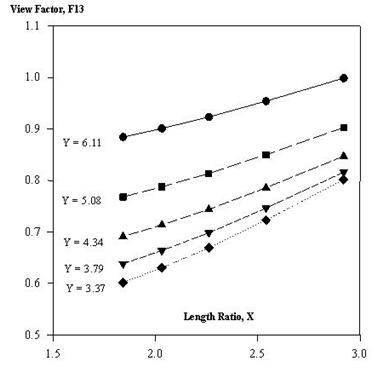Figure 3. Effect of varying length ratios on view factors for perpendicular plate geometry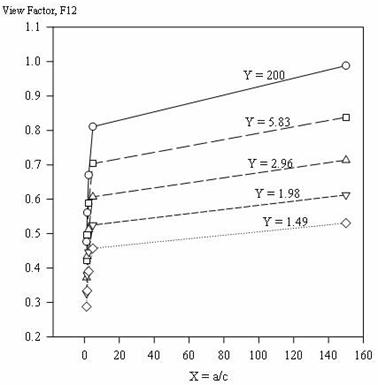Figure 2. Effect of varying length ratios on view factors for identical, parallel plate geometry

Conclusions

Computer programs have been developed to calculate view factors which can be used for the evaluation of radiation exchange within rectangular non-absorbing enclosures. These programs can therefore be a valuable aid in the development of mathematical models utilizing the zone method of analysis for radiant exchange. The results from this study are in good agreement with published data.

Furthermore, it became evidently clear that, the computer program for the numerical work can handle much more complex geometry/thermal systems within a small time interval; hence it is time saving as compared to the traditional graphical method. The algorithm is so designed such that variations in furnace operating conditions, properties, dimensions and temperatures, etc; are possible with only slight modifications in the main computer program. The method, therefore, allows for proper furnace analysis as regards the radiative heat transfer. It can also handle different furnace geometries.

References

 Bevans J. T., Dunkle R. V., Radiant Interchange Within an Enclosure, Journal of Heat Transfer, Trans. ASME, Series C, 1960, vol. 82, p. 1-19.

 Gray W. A., Muller R., Engineering Calculations in Radiative Heat Transfer, Pergamon Press Ltd., 1974, vol. 13, p. 1-75.

 Hottel H. C., Sarofim A. F., Radiative Transfer, Mcgraw-Hill, New York, 1967.

 Kreith F., Principles of Heat Transfer, IEP-A DUN-DONNELLEY, 3rd Edition, pp. 219-281, 1976.

 Sparrow E. M., Radiant Interchange Between Surfaces by Non-absorbing and Non-emitting Media, Handbook of Heat Transfer, Mc Graw-Hill, pp. 15 & 43-51, 1973.